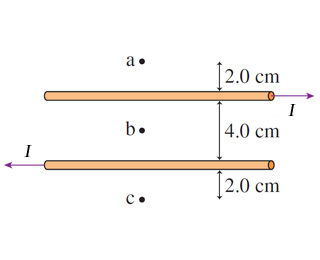# Problem: Assume that I = 11 A .Part AWhat is the magnetic field strength at point a? Express your answer to two significant figures and include the appropriate units.Part BWhat is the direction of the magnetic field at point a?

###### FREE Expert Solution

Magnetic field around a conductor:

$\overline{){\mathbf{B}}{\mathbf{=}}\frac{{\mathbf{\mu }}_{\mathbf{0}}\mathbf{i}}{\mathbf{2}\mathbf{\pi }\mathbf{r}}}$

Right-hand grip rule: The thumb points in the direction of the current, fingers curl in the direction of the magnetic field.

(a)

Using the right-hand rule, the magnetic field at a due to the top wire is out of the page. The magnetic field due to the lower wire is into the page.

93% (118 ratings)###### Problem Details

Assume that I = 11 A .Part A

What is the magnetic field strength at point a? Express your answer to two significant figures and include the appropriate units.

Part B

What is the direction of the magnetic field at point a?